# Easiest colleges to get into in Wisconsin

Top 10 colleges in Wisconsin with the highest acceptance rates
What are the easiest colleges to get into in Wisconsin? We've got you covered. We've compiled a national college database and have created a list of the easiest colleges to get into in Wisconsin below. These are the easiest 4 year colleges to get into in Wisconsin that have the highest acceptance rates, and are more likely to accept low GPAs, GEDs, and everything in between. There are tons of good colleges below that accept more applicants, and we also share their average SAT and ACT scores so that you can see where you're most competitive. Read on to find out more.

## University of Wisconsin Green Bay acceptance rate

University of Wisconsin Green Bay acceptance rate is 92.5%.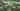The average SAT score for University of Wisconsin Green Bay is 1110.

• SAT composite: 1110
• SAT math: 555

The average ACT score for University of Wisconsin Green Bay is 22.

## Wisconsin Lutheran College acceptance rate

Wisconsin Lutheran College acceptance rate is 90.3%.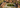The average SAT score for Wisconsin Lutheran College is 1152.

• SAT composite: 1152
• SAT math: 570

The average ACT score for Wisconsin Lutheran College is 23.

## University of Wisconsin Stout acceptance rate

University of Wisconsin Stout acceptance rate is 86%.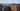The average SAT score for University of Wisconsin Stout is 1110.

• SAT composite: 1110
• SAT math: 555

The average ACT score for University of Wisconsin Stout is 22.

## University of Wisconsin Eau Claire acceptance rate

University of Wisconsin Eau Claire acceptance rate is 86%.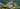The average SAT score for University of Wisconsin Eau Claire is 1150.

• SAT composite: 1150
• SAT math: 580

The average ACT score for University of Wisconsin Eau Claire is 24.

## University of Wisconsin Whitewater acceptance rate

University of Wisconsin Whitewater acceptance rate is 83.2%.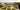The average SAT score for University of Wisconsin Whitewater is 1100.

• SAT composite: 1100
• SAT math: 560

The average ACT score for University of Wisconsin Whitewater is 22.

## Marquette University acceptance rate

Marquette University acceptance rate is 81.7%.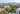The average SAT score for Marquette University is 1240.

• SAT composite: 1240
• SAT math: 620

The average ACT score for Marquette University is 27.

## University of Wisconsin Stevens Point acceptance rate

University of Wisconsin Stevens Point acceptance rate is 81%.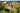The average SAT score for University of Wisconsin Stevens Point is 1040.

• SAT composite: 1040
• SAT math: 510

The average ACT score for University of Wisconsin Stevens Point is 23.

## University of Wisconsin Platteville acceptance rate

University of Wisconsin Platteville acceptance rate is 79.1%.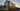The average SAT score for University of Wisconsin Platteville is 1245.

• SAT composite: 1245
• SAT math: 630

The average ACT score for University of Wisconsin Platteville is 24.

## Cardinal Stritch University acceptance rate

Cardinal Stritch University acceptance rate is 78.3%.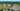The average SAT score for Cardinal Stritch University is 1033.

• SAT composite: 1033
• SAT math: 530

The average ACT score for Cardinal Stritch University is 21.

## University of Wisconsin La Crosse acceptance rate

University of Wisconsin La Crosse acceptance rate is 78.2%.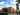The average SAT score for University of Wisconsin La Crosse is 1174.

• SAT composite: 1174
• SAT math: 590